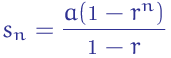# Geometric Series Formula

The Geometric series formula or the geometric sequence formula gives the sum of a finite geometric sequence. The geometric series is that series formed when each term is multiplied by the previous term present in the series. The sequence will be of the form {a, ar, ar2, ar3, …….}.

## Geometric Series Formula

The geometric series formula is given byHere a will be the first term and r is the common ratio for all the terms, n is the number of terms.

### Solved Example Questions Based on Geometric Series

Let us see some examples on geometric series.

Question 1: Find the sum of geometric series if a = 3, r = 0.5 and n = 5.

Solution:

Given:

a = 3

r = 0.5

n = 5

sn =

$$\begin{array}{l}\frac{a(1-r^n)}{1-r}\end{array}$$

The sum of five terms is given by S5=

$$\begin{array}{l}\frac{3(1- (0.5)^5)}{1- 0.5}\end{array}$$

= 5.8125

Question 2: Find S10 if the series is 2, 40, 800,…..

Solution:

From the given,

a = 2

r = 20

n = 10

The 10th term in the series is given by S10 =

$$\begin{array}{l}\frac{a(1-r^n)}{1-r} = \frac{2(1-20^{10})}{1-20}\end{array}$$

=

$$\begin{array}{l}\frac{2(1-20^{10})}{1-20} = \frac{2 \times (-1.024 \times 10^{13})}{-19}\end{array}$$

=

$$\begin{array}{l}\frac{-2.048 \times 10^{13}}{-19}\end{array}$$
= 1.0778 × 1012.